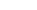•WE PROVIDECALCULUS
HOMEWORK
HELP
We can help with
Continuity
Functions
Derivatives
and Integration
starts at \$25/hour.

# Calculus Homework Help

• Functions
• Polynomials
• Rational Functions
• Logarithms
• Exponentials
• Trigonometric

• Limits
• Direct Substitution Property
• Undetermined Forms
• Trigonometric Limits
• Factorization
• Side Limits
• L'Hopital Rule

• Continuity
• Connection with limits
• Algebraic Properties
• Intermediate Value Theorem

• Derivatives
• Tangent Line
• Product Rule
• Quotient Rule
• Chain Rule
• Related Rates
• Mean Value Theorem
• Maximum and Minimum values

• Integrals
• Antiderivatives
• Fundamental Theorem of Calculus
• Substitutions and change of variables
• Partial Fractions
• Improper Integrals

• Series
• Positive Series
• Convergence criteria
• Alternating Series

## Claim Your Free Solved Stats Problems!

* indicates required
Email Format

## Our Specialties

#### All Calculus Subjects

We can help you with ALL Calculus subjects. We know that Calculus is hard topic, and we can provide the kind of help you need, with the kind of expert who will understand immediately what needs to get done.

#### Differentiation

Are you stuck calculating a derivative that uses the Chain Rule, Multiplication Rule and Quotient rule, all together?? We can help you with that. Our tutors can solve any kind of derivatives and related rates question.

#### Function Graphing

One of the tasks you may be faced in your Calculus class is that of graphing a function in detail. That involves, getting intercepts, asymptotes, maxima and minima, inflection points and a very accurate of all these elements together. We can help you that too.

#### Integration

This one can be a toughie for many. Integration may require advanced understanding of several Calculus topics, and may require of a great deal of creativity. We can help you with this too.

$\displaystyle \lim_{n\rightarrow \infty} \frac{2n^2+1}{3n^2-5n+2}$ $\displaystyle\frac{2n^2+1}{3n^2-5n+2}$ $\displaystyle\frac{2n^2+1}{3n^2-5n+2}= \frac{2+\frac{1}{n^2}}{ 3-\frac{5}{n}+\frac{2}{n^2} }\rightarrow \frac{2}{3}$ $\displaystyle x^{2/3}+y^{2/3}=a^{2/3}, \qquad a>0$ $\displaystyle \frac{2}{3} x^{-1/2}+\frac{2}{3} y^{-1/3} y'=0$ $\displaystyle x^{-1/2}+ y^{-1/3} y'=0$ $\displaystyle \frac{dy}{dx} = -\left(\frac{y}{x}\right)^{1/3}$ $\displaystyle x\left(\frac{dy}{dx}\right)^3=-y$ $\displaystyle \left(\frac{dy}{dx}\right)^3+3x\left(\frac{dy}{dx}\right)^2 y''=-y'$ $\displaystyle y''=\frac{y/x+(y/x)^{1/3}}{3x(y/x)^{2/3}}$ $\displaystyle y''=\frac{(y/x)^{2/3}+1}{3x(y/x)^{1/3}}=\frac{y^{2/3}+x^{2/3}}{3x^{4/3}y^{1/3}}$ $\displaystyle y''=\frac{a^{2/3}}{3x^{4/3}y^{1/3}}$